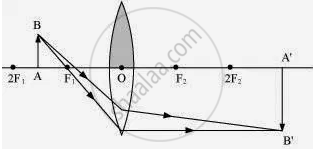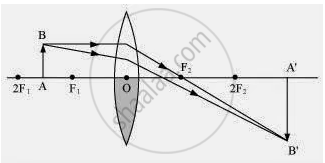# One-half of a convex lens is covered with a black paper. Will this lens produce a complete image of the object? Verify your answer experimentally. Explain your observations. - Science

One-half of a convex lens is covered with a black paper. Will this lens produce a complete image of the object? Verify your answer experimentally. Explain your observations.

One-half of a convex lens is covered with a black paper. Will such a lens produce an image of the complete object? Support your answer with a ray diagram.

#### Solution

The convex lens will form complete image of an object, even if its one half is covered with black paper. It can be understood by the following two cases.Case IWhen the upper half of the lens is covered
In this case, a ray of light coming from the object will be refracted by the lower half of the lens. These rays meet at the other side of the lens to form the image of the given object, as shown in the above figure.

Case IIWhen the lower half of the lens is covered
In this case, a ray of light coming from the object is refracted by the upper half of the lens. These rays meet at the other side of the lens to form the image of the given object, as shown in the above figure.

Concept: Convex Lens
Is there an error in this question or solution?

#### APPEARS IN

NCERT Class 10 Science
Chapter 10 Light – Reflection and Refraction
Q 9 | Page 186